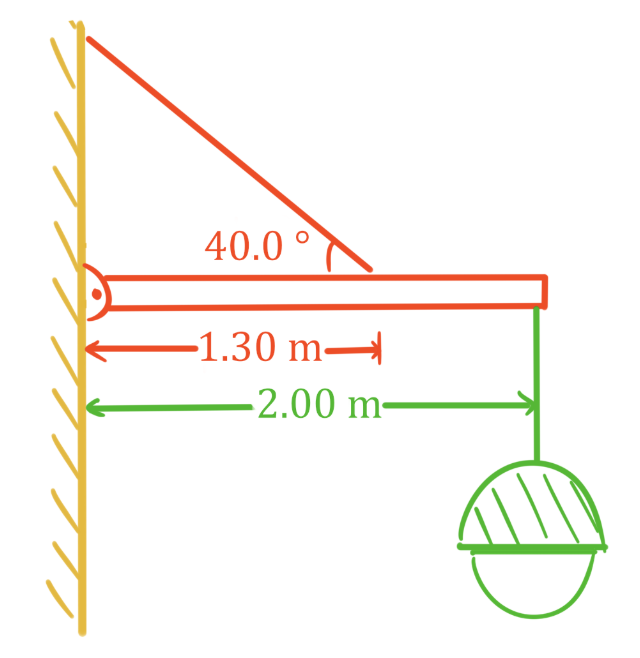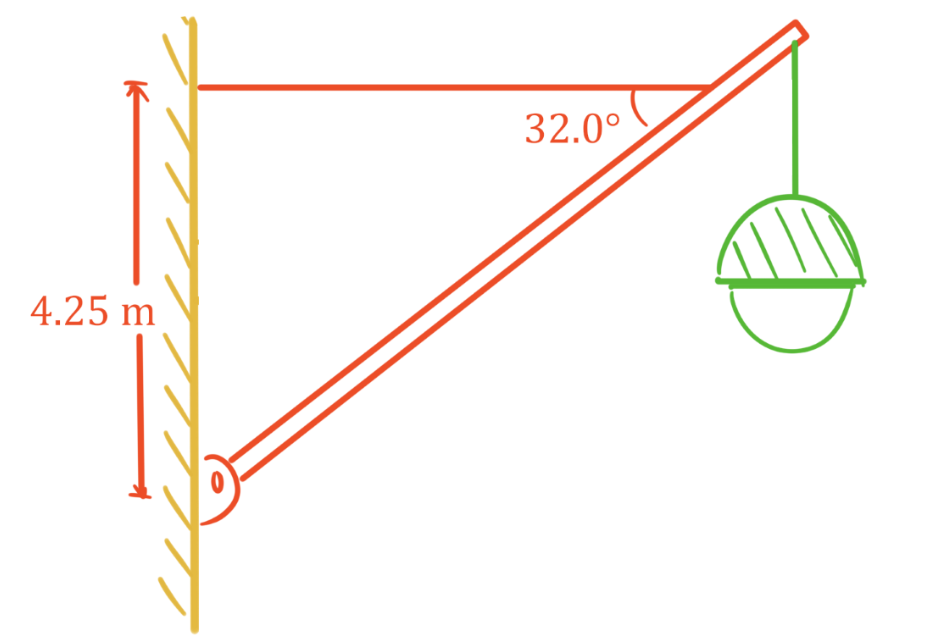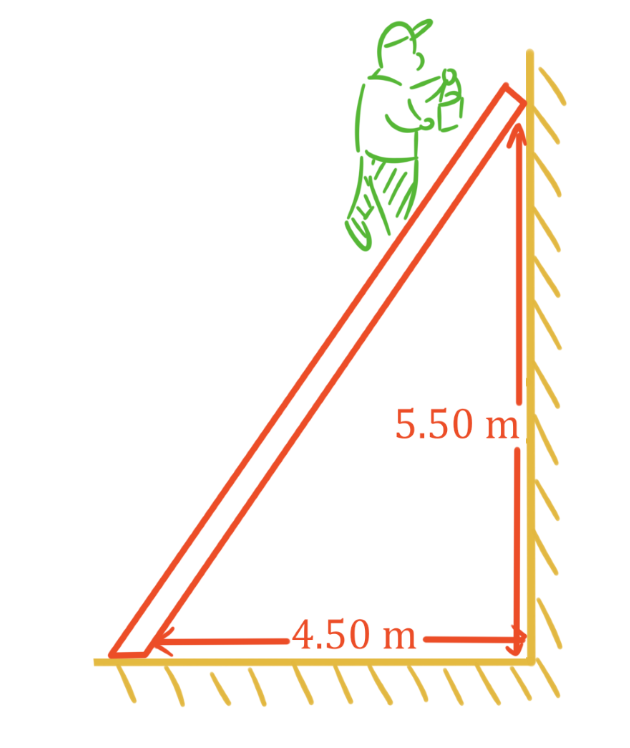# Static equilibrium problems

### Static equilibrium problems

#### Lessons

In this lesson, we will learn:

• Solving statics problems using both translational and rotational equilibrium

Notes:

• An object or group of objects that are not moving are in static equilibrium.
• In static equilibrium, the conditions for both translational and rotational equilibrium must be met.
Conditions for Translational Equilibrium

$\Sigma F = 0 N$

or equivalently:

$\Sigma F_{x} = 0 N$ and $\Sigma F_{y} = 0 N$

$\Sigma F:$ sum of all forces, in newtons (N)

$\Sigma F_{x}:$ sum of all force components in x direction, in newtons (N)

$\Sigma F_{y}:$ sum of all force components in y direction, in newtons (N)

Torque

$\tau = F_{\perp}d$

$\tau$: torque, in newton meters (N·m)

$F_{\perp}:$ component of force perpendicular to $d$, in newtons (N)

$d:$ distance from point of rotation, in meters (m)

Conditions for Rotational Equilibrium

$\Sigma \tau = 0$ N·m

or simpler equation:

total $CW$ $\tau$ = total $CCW$ $\tau$

$\Sigma \tau :$ sum of all torques, in newton meters (N·m)

total $CW$ $\tau$: magnitude of all torques in the clockwise direction, in newton meters (N·m)

total $CCW$ $\tau$: magnitude of all torques in the counterclockwise direction, in newton meters (N·m)

• 1.
Cable and beam in static equilibrium
a)
A 20.0 kg lamp is hung from a uniform 12.5 kg beam as shown. Find the tension in the wire, and the horizontal and vertical forces acting on the hinge.b)
A 25.0 kg lamp is hung from an 18.0 kg uniform beam as shown. The total length of the beam is 8.50 m. Find the tension in the wire, and the horizontal and vertical forces acting on the hinge.• 2.
Ladder beginning to slide

An 80.0 kg painter climbs 85% of the way up a uniform 25.0 kg ladder before it starts to slip backwards along the ground. What is the coefficient of static friction between the ladder and ground? Assume the wall is frictionless.